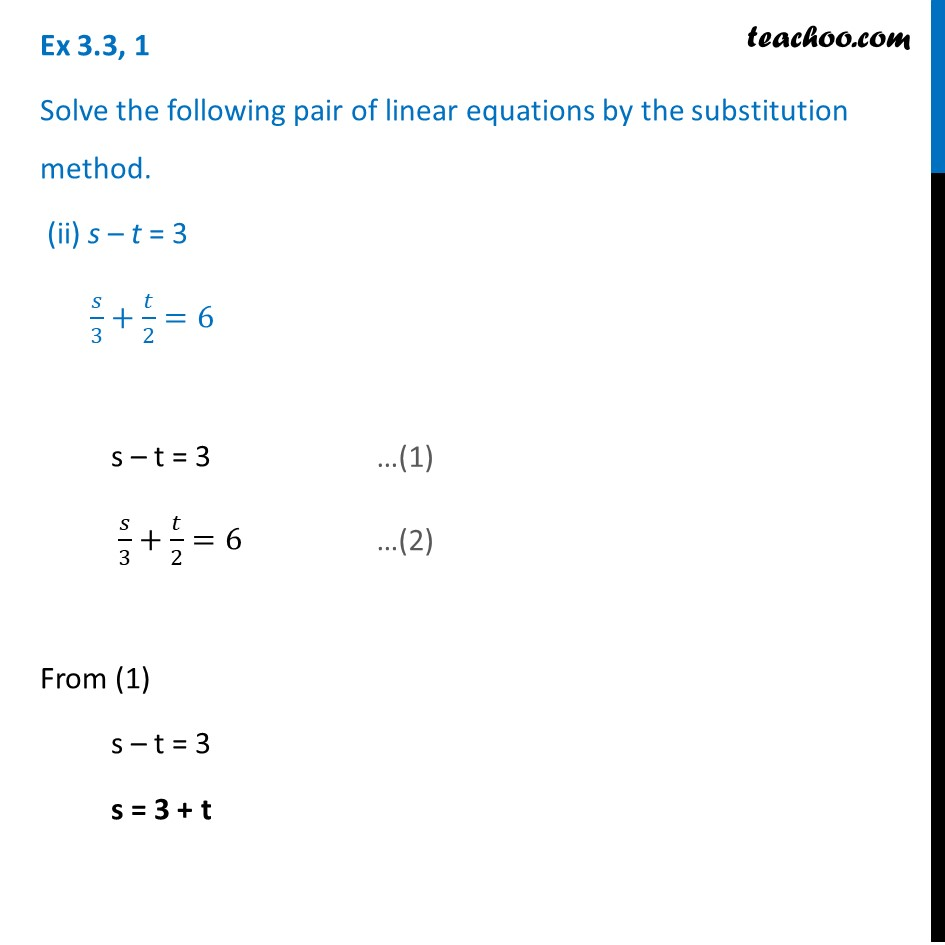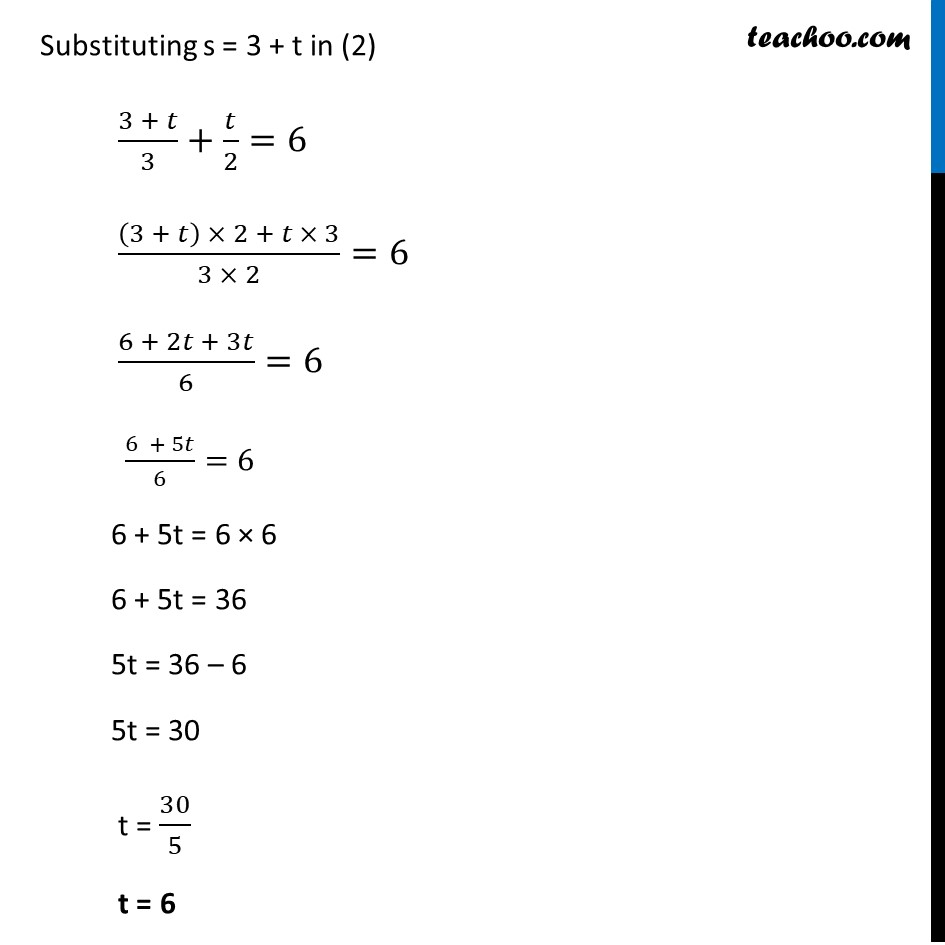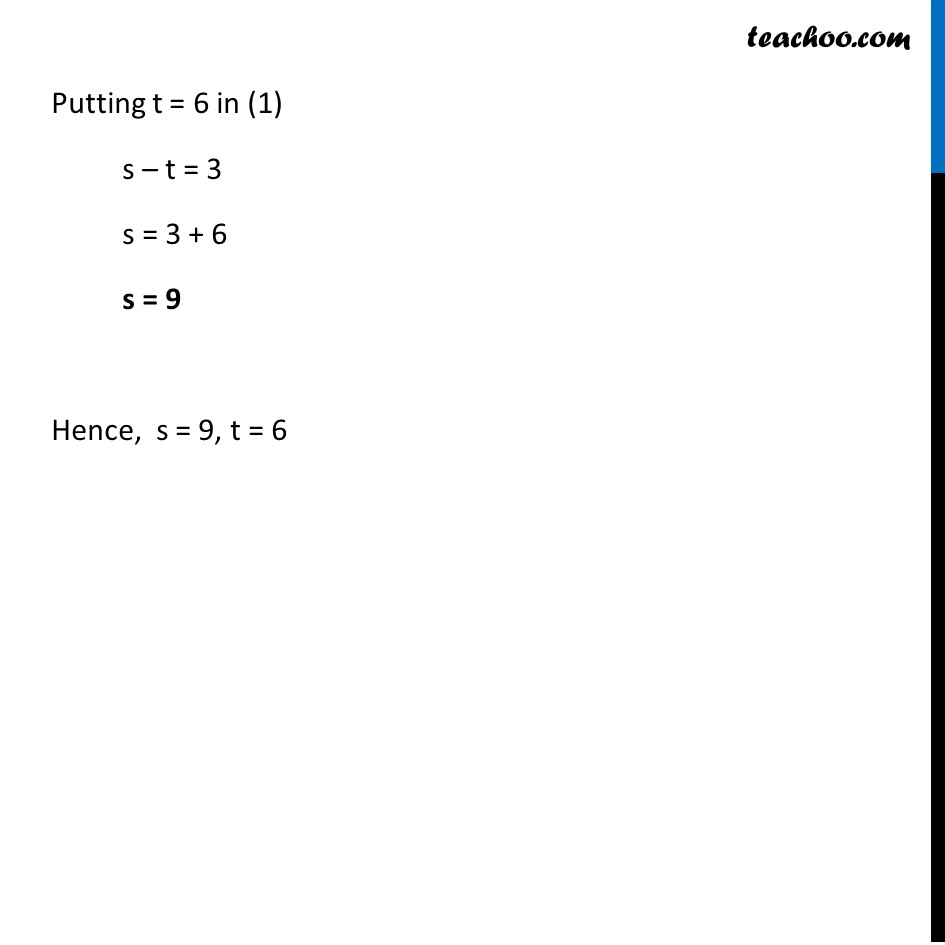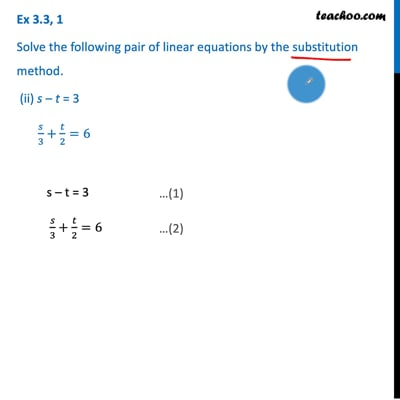Ex 3.3

Chapter 3 Class 10 Pair of Linear Equations in Two Variables
Serial order wiseThis video is only available for Teachoo black users

Get live Maths 1-on-1 Classs - Class 6 to 12

### Transcript

Ex 3.3, 1 Solve the following pair of linear equations by the substitution method. (ii) s – t = 3 𝑠/3+𝑡/2=6 s – t = 3 𝑠/3+𝑡/2=6 From (1) s – t = 3 s = 3 + t Substituting s = 3 + t in (2) (3 + 𝑡)/3+𝑡/2=6 ((3 + 𝑡) × 2 + 𝑡 × 3)/(3 × 2)=6 (6 + 2𝑡 + 3𝑡)/6=6 (6 + 5𝑡)/6=6 6 + 5t = 6 × 6 6 + 5t = 36 5t = 36 – 6 5t = 30 t = 30/5 t = 6 Putting t = 6 in (1) s – t = 3 s = 3 + 6 s = 9 Hence, s = 9, t = 6